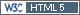## Kenward-Roger? [Study As­sess­ment]

Hi Angus,

» Jerry from NCSS reports the following:
»
» We have looked into this and resolved it in our own minds. NCSS uses the Kenwood-Rogers method for degrees of freedom which is an extension of the Satterthwaite method.

Really? Still I can’t reproduce results of NCSS in R (data prepared like in this post).

```library(lmerTest) library(pbkrtest) muddle   <- lmer(log(Cmax) ~ log(Dose) + (1|Subj), data=resp) sum.Satt <- summary(muddle, ddf="Satterthwaite") res.Satt <- data.frame(0:1, sum.Satt\$coeff[ , "Estimate"],                        sum.Satt\$coeff[, 2],                        sum.Satt\$coeff[, 3],                        sum.Satt\$coeff[, 1]-                        qt(1-0.05, sum.Satt\$coeff[, 3])*                        sum.Satt\$coeff[, 2],                        sum.Satt\$coeff[, 1]+                        qt(1-0.05, sum.Satt\$coeff[, 3])*                        sum.Satt\$coeff[, 2],                        row.names=NULL) sum.KR <- summary(muddle, ddf="Kenward-Roger") res.KR <- data.frame(0:1, sum.KR\$coeff[ , "Estimate"],                      sum.KR\$coeff[, 2],                      sum.KR\$coeff[, 3],                      sum.KR\$coeff[, 1]-                      qt(1-0.05, sum.KR\$coeff[, 3])*                      sum.KR\$coeff[, 2],                      sum.KR\$coeff[, 1]+                      qt(1-0.05, sum.KR\$coeff[, 3])*                      sum.KR\$coeff[, 2],                      row.names=NULL) names(res.KR) <- names(res.Satt) <- c("B", "PE", "SE", "df",                                       "CLlower", "CLupper") print(round(res.Satt, 4), row.names=FALSE) print(round(res.KR, 4), row.names=FALSE)```

Satterthwaite
```B   PE     SE     df   CLlower CLupper 0 1.9414 0.2431 9.1956  1.4968  2.3860 1 0.7617 0.0473 5.8961  0.6696  0.8539```
Kenward-Roger
```B   PE     SE     df   CLlower CLupper 0 1.9414 0.2431 9.0915  1.4962  2.3866 1 0.7617 0.0473 5.7527  0.6692  0.8543```

Modified results of NCSS from a previous posts:
```B   PE     SE     df   CLlower CLupper 0 1.9414 0.2496 9.2     1.4849  2.3978 1 0.7617 0.0492 5.9     0.6659  0.8576```

As zizou suspected in this post there are differences in the SEs (therefore, we get different CIs even if DFs are identical) – which leaves the question open why they are different when compared to the other packages. NCCS seems to use Satterthwaite’s DFs and not Kenward-Roger’s (contrary to the documentation and what Jerry told you).

Cheers,
Helmut SchützThe quality of responses received is directly proportional to the quality of the question asked. ☼
Science QuotesIng. Helmut Schütz